### Home > CALC > Chapter 2 > Lesson 2.3.3 > Problem2-125

2-125.
1. Determine if the following functions are even, odd, or neither. Explain how you determined your choice. Homework Help ✎

1. y = sin2 x

2.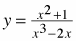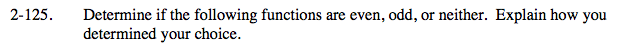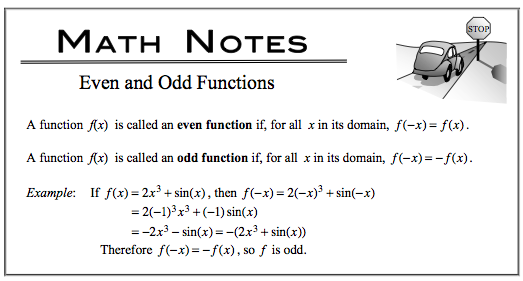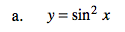y = sin2x = (sinx)(sinx)

y = sinx is an odd function. Definition of odd functions: f(−x) = −f(x) Therefore, sin(−x) = −sin(x).

Explore what y = sin2(−x) looks like:
y = sin2(−x)
= (sin(−x))(sin(−x))
=(−sinx)(−sinx)
= sin2x

Hence sin2(−x) = sin2(x).
This is the definition of an even function: f(−x) = f(x).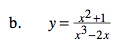Test for even: f(a) = f(−a)

$f(a)=\frac{a^{2}+1}{a^{3}-2a}$

$f(-a)=\frac{(-a)^{2}+1}{(-a)^{3}-2(-a)}=\frac{a^{2}+1}{-a^{3}+2a}\ ,$

f(a) ≠ f(−a); not even

Test for odd: f(−a) = −f(a)...